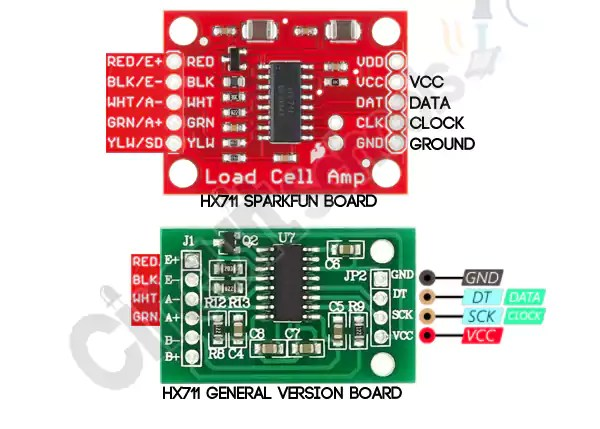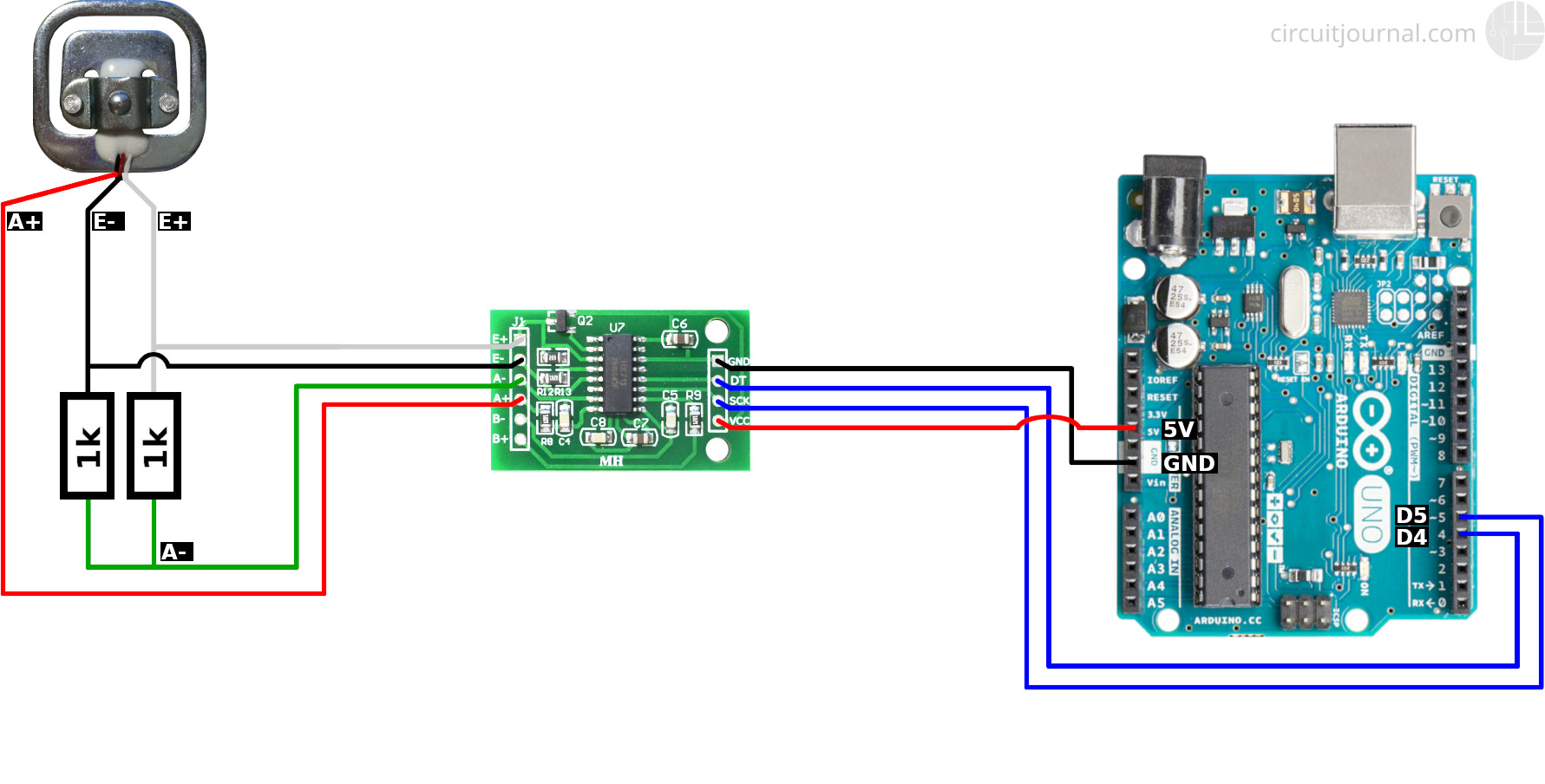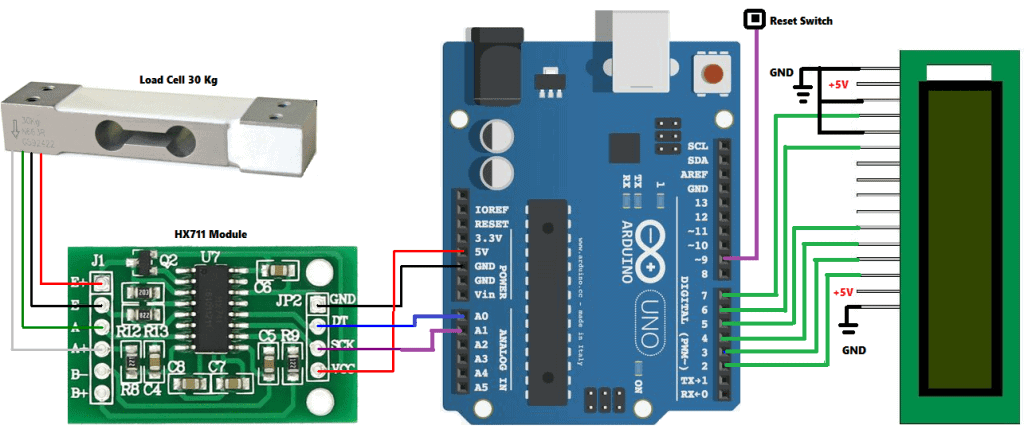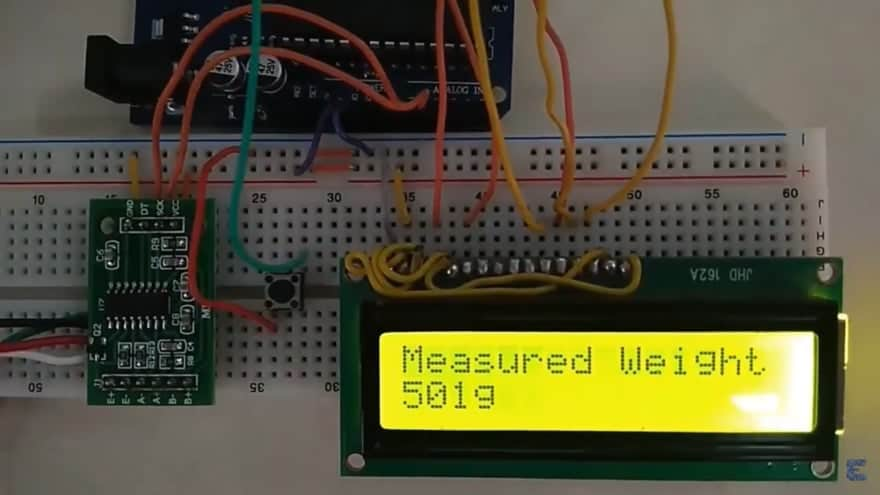# How to Use Arduino Strain Gauge Circuits for Accurate Measurement

Strain gauge circuits are essential in many projects, providing accurate measurement results to engineers, scientists and technicians. Arduino makes it easy to build a strain gauge circuit with its powerful microcontrollers and digital signal processing capabilities. Using an Arduino strain gauge circuit allows you to measure both static and dynamic loads and accurately determine their weight or force. This article provides an overview of how to use Arduino strain gauge circuits, including wiring diagrams and helpful tips.

Arduino is an open-source electronics platform based on easy-to-use hardware and software. The combination of microcontrollers, sensors, and programming makes it possible to design and create professional-level projects. Arduino strain gauge circuits are one example of the many ways that Arduino can be used for practical purposes. By providing an accurate readout of a strain gauge's output, these circuits can measure static and dynamic forces in a wide range of applications.

## Understanding Strain Gauges and Their Principles of Operation

A strain gauge is an electrical device used to measure strain. It works by sensing the changes in resistance of a circuit when a load is applied. Strain gauges are commonly used in a variety of industrial and scientific applications, such as bridge integrity testing and geotechnical investigations.

A strain gauge consists of a small metal foil that is attached to a substrate material. When a load is applied to the strain gauge, the metal foil deforms and changes its resistance. This change in resistance is detected by a Wheatstone bridge, which is an electronic circuit consisting of four resistors arranged in a square configuration. The output of the Wheatstone bridge is then sent to an amplifier, which amplifies the signal and sends it to the Arduino for further processing.

## Setting Up an Arduino Strain Gauge Circuit

The diagram below illustrates the typical setup for an Arduino strain gauge circuit.

![alt text](https://i.stack.imgur.com/U5m6U.png "Arduino Strain Gauge Circuit Diagram")

In the diagram, the strain gauge is connected to the Wheatstone bridge. The output of the Wheatstone bridge is connected to the Arduino’s analog pins. The Arduino then uses its onboard ADC (Analog-to-Digital Converter) to convert the analog signal into a digital signal, which can then be processed.

The key components of an Arduino strain gauge circuit are:

• Strain Gauge

• Wheatstone Bridge

• Amplifier

• Arduino

In order to get accurate readings, the strain gauge must be properly calibrated. This is done by connecting a known load to the strain gauge and measuring the output voltage. Once the calibration is complete, the Arduino can be programmed to interpret the strain gauge’s readings.

## Tips for Optimizing an Arduino Strain Gauge Circuit

Here are some tips for optimizing an Arduino strain gauge circuit:

• Make sure the strain gauge is securely mounted and connected to the Wheatstone bridge.

• Use shielded cables to reduce noise and interference.

• Check the calibration of the strain gauge regularly.

• Use a high-quality amplifier to ensure accurate readings.

• Be sure to use an appropriate power supply for the strain gauge.

By following these tips, you can ensure that your Arduino strain gauge circuit is optimized for accurate measurements.

## Conclusion

Arduino strain gauge circuits provide accurate measurements of static and dynamic forces. By understanding how strain gauges work and setting up the Arduino strain gauge circuit correctly, engineers, scientists and technicians can optimize their systems for precise results. This article provides an overview of how to use Arduino strain gauge circuits, including wiring diagrams and helpful tips for optimization.Strain Gauge Bending Test Sensor Module Weight Voltage Output Dvr For Arduino 4 99 Picclick UkStrain Gauges Wheatstone Bridge Hx711 To Measure Values Project Guidance Arduino ForumStrain Es Wheat Stone Bridge Science And Measurement Arduino ForumStrain Gauge Module With Bf350 MakerfabsWeighing Scale Using Load Cell And Hx711 Amplifier With Arduino Circuit SchoolsStrain Es Wheat Stone Bridge Science And Measurement Arduino ForumSketch Drawing For The Strain Gauge Pull Meter With Its Wiring And Scientific DiagramStrain Gauge Connection Scientific DiagramArduino Hx711 To Get Strain Values Sensors ForumForce Measurement With Arduino And The Hx711 Module Aranacorp50kg Load Cells With Hx711 And Arduino 4x 2x 1x Diagrams Circuit JournalStrain Gauge Wheatstone Bridge Circuit Diagram Sensors Arduino ForumWeighing Machine Using Arduino Load Cell Hx711 ModuleArduino Interfacing With Stone St 056w 01 Strain Gauge Projectiot123 Technology Information Website WorldwideStrain Gauge Meter With Hx711 Sensors Arduino ForumStrain Es Wheat Stone Bridge Science And Measurement Arduino ForumArduino Weight Measurement Project With Load Cell And Hx711 Module Interfacing Circuit Diagram CodeInterfacing Load Cell With Arduino Using Hx711Weighing Machine Using Arduino Load Cell Hx711 Module